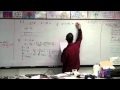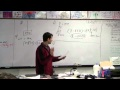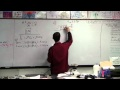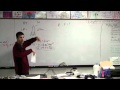Whatson G Forces Test .:. Mock test .:. Go Up

Cross off 1 page:

NSL - Newton's Second Law worksheet
NF - Net Force worksheet
F - Friction worksheet

Page 1-  Person pushing on stage set with and without friction.

1. What is the acceleration of the set - NSL #1
2. Final velocity (using linear kinematics formulas) - NSL #5
3. Conceptual question (answer using words) - how could you increase the acceleration? (Notes from lectures? - 01-01NewtonLaws.ppt)
4. Acceleration with two forces acting on the set - NF #9
5. Force to accelerate from rest to a velocity over a particular distance - NF #7

Page 2-  Crane lifting a load vertically

1. Tension when the load is not accelerating - NSL #8
2. Tension when the load is accelerating at down or up NF #10
3. Acceleration when the tension is a particular number - NF #4
4. Calculate the Tension when accelerating down or up - NF #10
5. Define in words: weight and mass, give units, and tell the difference between them - (look at PowerPoint lecture: 02-01MassVsWeight.ppt)

Page 3-  Red Elk's dogs sliding a sled. Friction: 4a, 7, 8

1. What is the weight of the sled? - NSL #8
2. What is the force to start the sled moving? - F #4a also look at PowerPoint lecture: 05-01Friction.ppt
3. What force to keep the sled moving? - F #4a also look at PowerPoint lecture: 05-01Friction.ppt
4. Acceleration a particular applied force - F #7
5. Force to cause a particular acceleration - F #9

Page 4-  Rocket taking off from earth.

1. Calculate from distance and time the acceleration - NF #8 - acceleration like that
2. Draw a free body diagram of the upward accelerating rocket, calculate the weight - Like lecture 03-01NetForce.ppt slide 12, and  NSL #8
3. Calculate the thrust of the engine - NF #8
4. Find the acceleration if the thrust is a particular number - NF #4
5. Conceptual question regarding inertia in a weightless environment. (Words)

Force Mock Test

1. A 467 gram air track glider rests on a frictionless surface.  A force of 2.1 N is exerted on it for a distance of 35 cm.

a. What is the glider’s acceleration and final velocity? (4.50 m/s/s, 1.77 m/s)
b. What force would accelerate the glider from rest to 3.1 m/s in 0.12 seconds?
(12.1 N)
c. If there had been a force of 0.7 N opposing the 2.1 N force, what would have been the final velocity?
(1.45 m/s)7:13 - Video of Murray solving this problem

2. A 3.4 kg object hangs on a string.

a. What is the tension in the string if the velocity is zero?  What if the velocity is a constant 1.2 m/s up? (33.32 N, 33.32 N)
b. What is the tension in the string if the mass is accelerating at 1.8 m/s/s down?
(27.2 N)
c. If there is a tension of 38 N in the string, what is its acceleration?
(1.38 m/s/s up)6:36 - Video of Murray solving this problem

3. A 2.7 kg box has a kinetic and static coefficient of friction with the floor of 0.12 and 0.35.

a. What force is needed to start it moving, and what to keep it moving at a constant speed? (3.18 N, 9.26 N)
b. What force would be necessary to accelerate the box once it is moving at 5.2 m/s/s in the direction it is moving?
(17.2 N)
c. What is the acceleration of the box if it is moving to the right, and there is a force of 2.6 N to the right?
(-0.213 m/s/s – it is slowing down)7:52 - Video of Murray solving this problem

4. A 24 kg rocket takes off from the surface of the earth and reaches a height of 52 m in 1.89 seconds

a. What is the rocket’s acceleration? (29.1 m/s/s)
b. What must be the upward force exerted by the engines?
(934 N)
c. What acceleration would an upward force of 430 N cause?
(8.12 m/s/s upward)7:47 - Video of Murray solving this problem